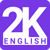# Latest MBA Aptitude Question SOLUTION: If f(x, y) = 1 + 2 + 3 + â€¦. + x if x > y and f(x, y) = 1 + 2 + 3 + â€¦. + y if y > x, then find the value of f(0, 1) + f(2, 1) + f(2, 3) + f(4, 3) + f(4, 5) + â€Launched an English app featuring 2000 mostly asked english words in all Competitive Exams. #2kenglish Download Now, It's Free